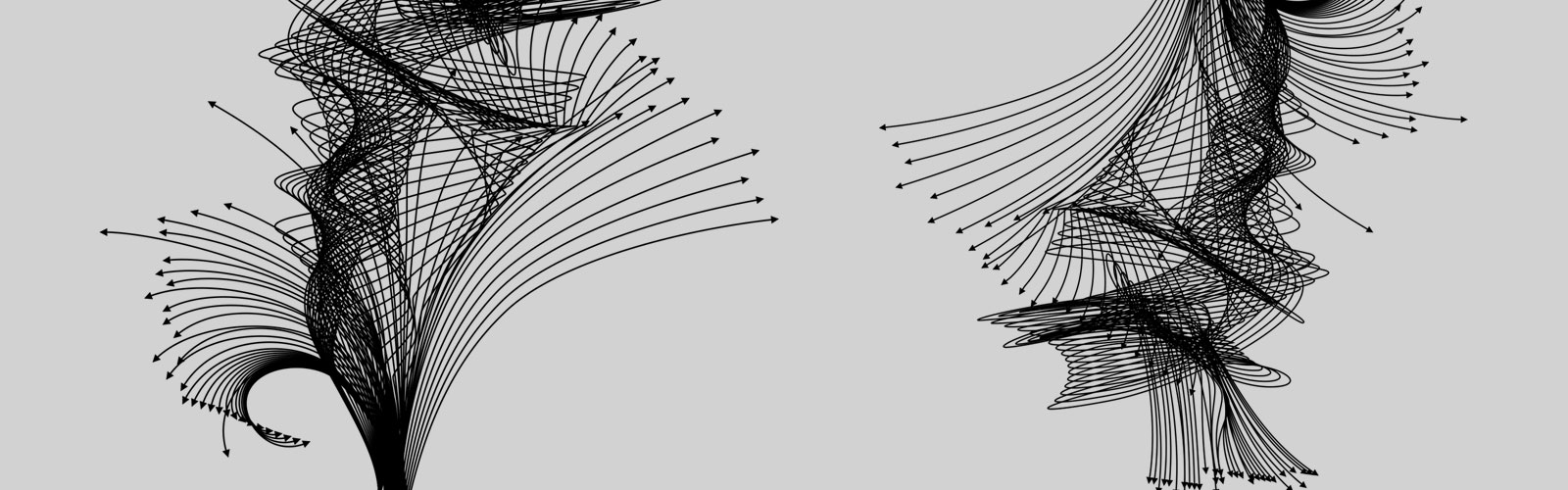Equivalence relations for ordinary differential equationsSpeaker:  Max Tschaikowski - IMT Lucca
Wednesday, November 16, 2016 at 5:00 PM rinfresco 16.45, inizio seminario 17.00
Ordinary differential equations (ODEs) are the primary means to modeling systems in a wide range of natural and engineering sciences. When the inherent complexity of the system under consideration is high, the number of equations required poses a serious detriment to our capability of performing effective analyses. This has motivated a large body of research, across many disciplines, into abstraction techniques that provide smaller ODE systems preserving the original dynamics in some appropriate sense.

In this talk I will review some recent results that look at this problem from a computer-science perspective. I will present equivalences for nonlinear ODEs that induce a quotienting of the set of ODE variables. This leads to a self-consistent reduced ODE system each macro-variable represents the dynamics of an equivalence class, either exactly or approximately with computable bounds.

For a rather general class of nonlinear ODEs, computing the largest equivalences can be done using a symbolic partition-refinement algorithm that exploits an encoding into a satisfiability (modulo theories) problem. For ODEs with polynomial derivatives of degree at most two, covering the important cases of affine systems and chemical reaction networks, the partition refinement is based on Paige and Tarjan’s seminal solution to the coarsest refinement problem. This gives an efficient algorithm that runs in O(m n logn) time, where m is the number of monomials and n is the number of ODE variables.

I will present numerical evidence of effective reductions in realistic models from chemistry, systems biology, electrical engineering, and structural mechanics.

(Joint work with Luca Cardelli, Mirco Tribastone, and Andrea Vandin)

Place
Ca' Vignal 2, Floor 1, Lecture Hall M

Contact person
Peter Michael Schuster

Publication date
September 8, 2016